Search this Site

# Stock Status and Conservation Information(From ISC23 Plenary Report)

## Albacore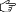Click here to see the ISC22 stock status and conservation information.

### Stock Status

Estimated summary biomass (males and females at age-1+) declined at the beginning of the time series until 2004 (Figure 1A). Subsequently, the summary biomass fluctuated without a trend until 2018, after which the biomass rapidly increased to historically high levels. It should be noted that the high summary biomass estimates during 2018-2021 were highly uncertain and should be treated with caution (Figure 1A). These high summary biomass estimates were due to historically high recruitment estimates in 2017 (~433 million fish; 95% CI: 194 – 671 million fish) (Figure 1C). However, recruitment estimates in the last 5 years (2017-2021) were highly uncertain and should be treated with caution. Estimated female SSB exhibited a similar population trend to the summary biomass, albeit with a lag of several years, and showed an initial decline until 2007 followed by fluctuations without a clear trend through 2021 (Figure 1B).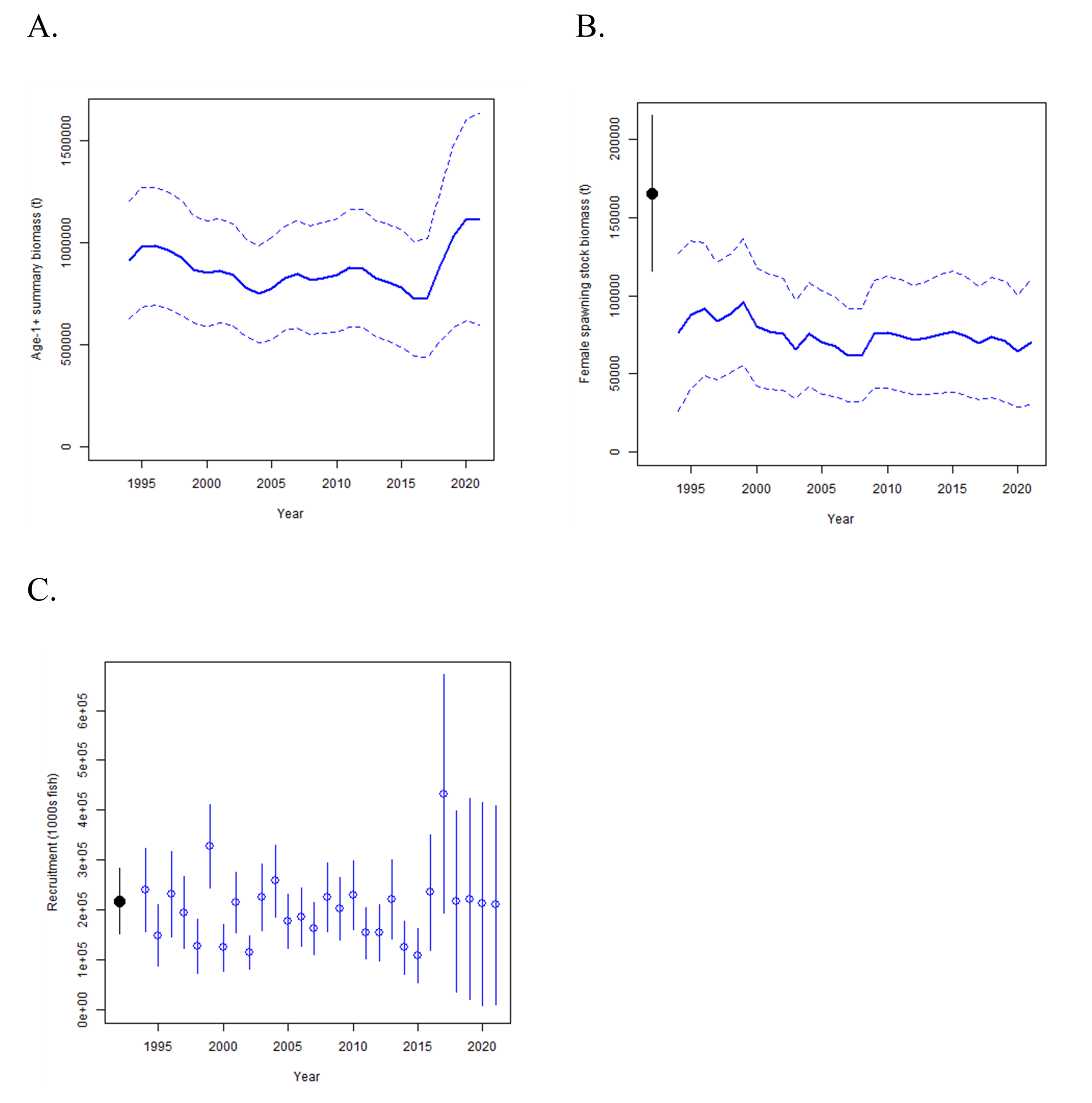Figure 1. Maximum likelihood estimates of (A) age-1+ biomass (B), female spawning biomass (SSB), and (C) age-0 recruitment of NPO ALB (Thunnus alalunga). Dashed lines (A and B) and vertical bars (C) indicate 95% confidence intervals. Closed black circle and error bars in (B) and (C) are the maximum likelihood estimate and 95% confidence intervals of unfished female spawning biomass, SSB0, and unfished recruitment, respectively, at equilibrium.

The average fishing intensity during 2018-2020 was estimated to be F59%SPR (95% CI: F72%SPR – F46%SPR), which was relatively moderate and resulted in a population with an SPR of approximately 59%. Instantaneous fishing mortality at age (F-at-age) was similar in both sexes through age-5, peaking at age-4 and declining to a low at age-6, after which males experienced higher F-at-age than females up to age 12 (Figure 2). Juvenile albacore aged 2 to 4 years comprised approximately 64% of the annual catch-at-age in numbers between 1994 and 2021 (Figure 3) due to the larger impact of surface fisheries (primarily troll, pole-and-line), which remove juvenile fish, relative to longline fisheries, which primarily remove adult fish (Figure 4).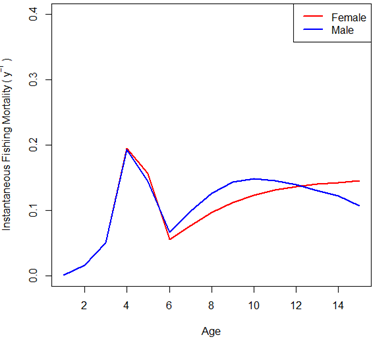Figure 2. Estimated sex-specific instantaneous fishing mortality-at-age (F-at-age) for the 2023 base case model, averaged across 2018-2020.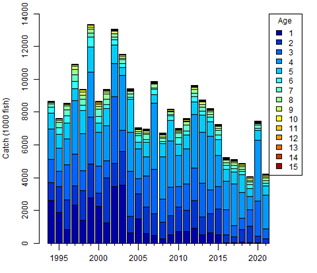Figure 3. Historical catch-at-age of NPO ALB (Thunnus alalunga) estimated by the 2023 base case model.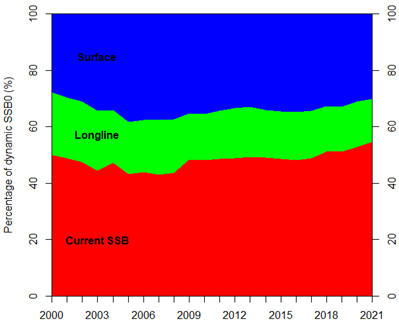Figure 4. Fishery impact analysis on NPO ALB (Thunnus alalunga) showing female spawning biomass (SSB) (red) estimated by the 2023 base case model as a percentage of dynamic, unfished female SSB (SSBcurrent, F=0). Colored areas show the relative proportion of fishing impact attributed to longline (green) and surface (blue) fisheries (primarily troll and pole-and-line gear but including all other gears except longline).

Stock status is depicted in relation to the target (F45%SPR), threshold (30%SSBcurrent, F=0), and limit (14%SSBcurrent, F=0) reference points (Figure 5A – the “La Jolla” plot; Table 1). The estimated female SSB has never fallen below the threshold and limit reference points since 1994, albeit with large uncertainty in the terminal year (2021) estimates. However, the estimated fishing intensity for five years (1999, 2002, 2003, 2004, and 2007) exceeded the target reference point. Even when alternative hypotheses about key model uncertainties such as growth were evaluated, the point estimate of female SSB in 2021 (SSB2021) did not fall below the threshold and limit reference points, although the risk increases with the more extreme assumption (Figure 5B). In contrast, estimated average fishing intensity during 2018-2020 (F2018-2020) did exceed the target reference point under one of these alternative hypotheses but did not exceed the average fishing intensity during the 2002-2004 period (Figure 5B; Table 1).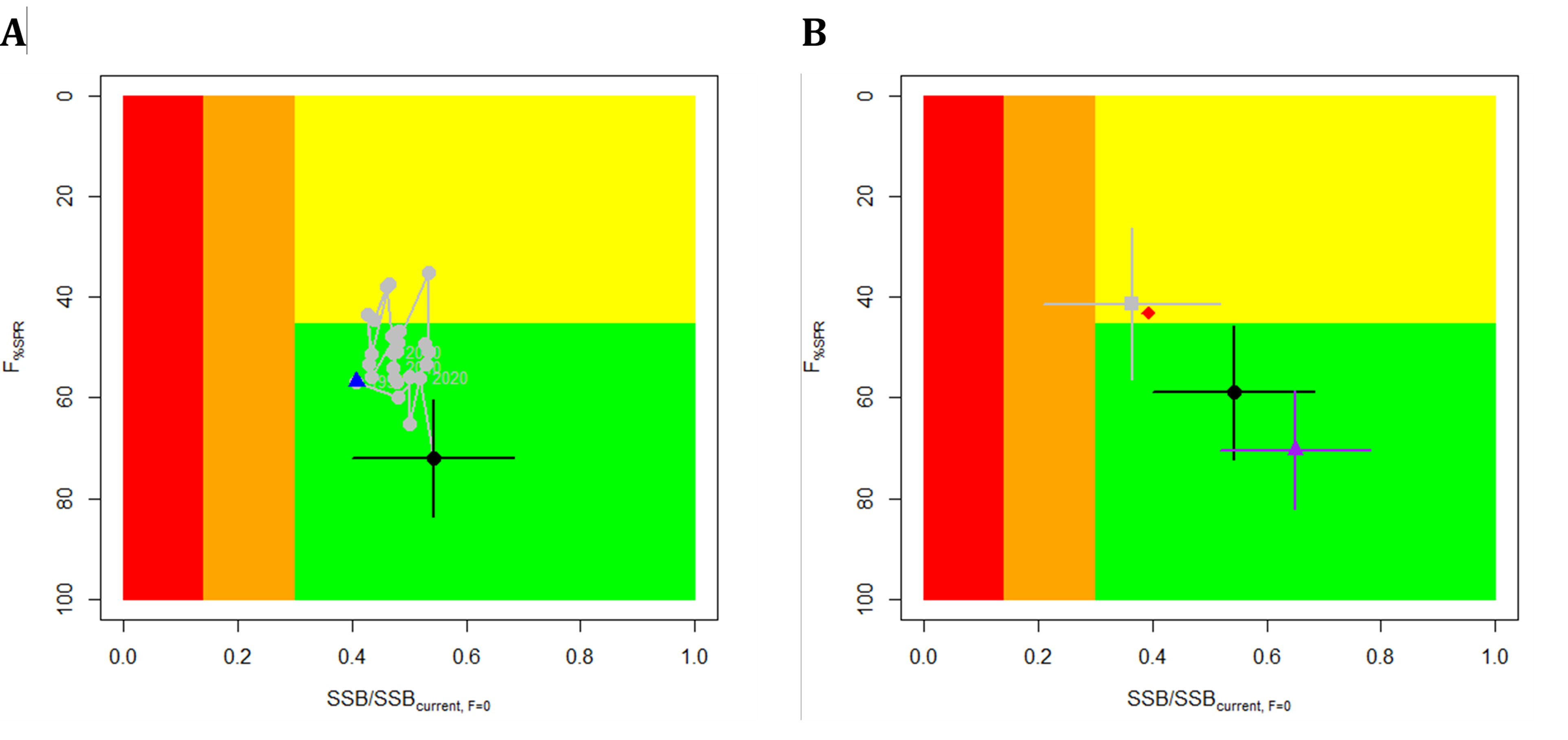Figure 5. (A) Stock status phase plot showing the status of the NPO ALB (Thunnus alalunga) stock relative to the biomass-based threshold (30%SSBcurrent, F=0) and limit (14%SSBcurrent, F=0) reference points, and fishing intensity-based target reference point (F45%SPR) over the modeling period (1994 – 2021). Blue triangle indicates the start year (1994) and black circle with 95% confidence intervals indicates the terminal year (2021). (B) Stock status plot showing current stock status and 95% confidence intervals of the base case model (black circle), an important sensitivity run of CV = 0.06 for Linf in the growth model (gray square), an important sensitivity run with an estimated growth model (purple triangle), and a model representing an update of the 2020 base case model to 2023 data (red diamond). 95% confidence intervals are not shown for the update of the 2020 base case model (red diamond) because the model did not have a positive definite Hessian matrix and uncertainty estimates were unreliable. Red zones in both panels indicate female SSBs falling below the limit reference point while the orange zones indicate female SSBs between the threshold and limit reference points. Green zones indicate female SSBs above the threshold reference point and fishing intensity levels below the target reference point. Yellow areas indicate female SSBs above the threshold reference point and fishing intensity levels above the target reference point. The Fs in this figure are indicators of fishing intensity based on spawning potential ratio (SPR) and calculated as %SPR. SPR is the ratio of the equilibrium SSB per recruit that would result from the estimated F-at-age relative to that of an unfished population. A higher %SPR indicates lower fishing intensity. Current fishing intensity values and SSB/SSBcurrent,F=0 ratios in (B) were calculated as the average during 2018-2020 (F%SPR, 2018-2020) and 2021 (SSB2021/SSBcurrent, F=0), respectively. The model representing an update of the 2020 base case model is similar to but not identical to the 2020 base case model due to changes in data preparation and model structure.Table 1. Estimates of maximum sustainable yield (MSY), female spawning stock biomass (SSB), fishing intensity (F), and reference point ratios for NP ALB for: 1) the base case model; 2) two important sensitivity models due to uncertainty in growth parameters; and 3) a model representing an update of the 2020 base case model to 2023 data. SSB0, SSBcurrent, F=0 and SSBMSY are the expected female SSB of a population in the equilibrium, unfished state; in the current, dynamic, unfished state; and at MSY, respectively. The Fs in this table are indicators of fishing intensity based on spawning potential ratio (SPR) and calculated as %SPR. SPR is the ratio of the equilibrium SSB per recruit that would result from the estimated F-at-age relative to that of an unfished population. Depletion is calculated as the proportion of the age-1+ biomass during the specified period relative to an unfished age-1+ equilibrium biomass. The model representing an update of the 2020 base case model is similar to but not identical to the 2020 base case model due to changes in data preparation and model structure.

The SSB2021 was estimated to be approximately 54% (95% CI: 40 – 68%) of SSBcurrent, F=0 and 1.8 (95% CI: 1.3 – 2.3) times greater than the estimated threshold reference point (Figure 6; Table 1). The estimated current fishing intensity (F2018-2020) was estimated to be F59%SPR (95% CI: F72%SPR – F46%SPR) and was lower than both the F45%SPR target reference point and the average fishing intensity during the 2002-2004 period (Figure 6; Table 1).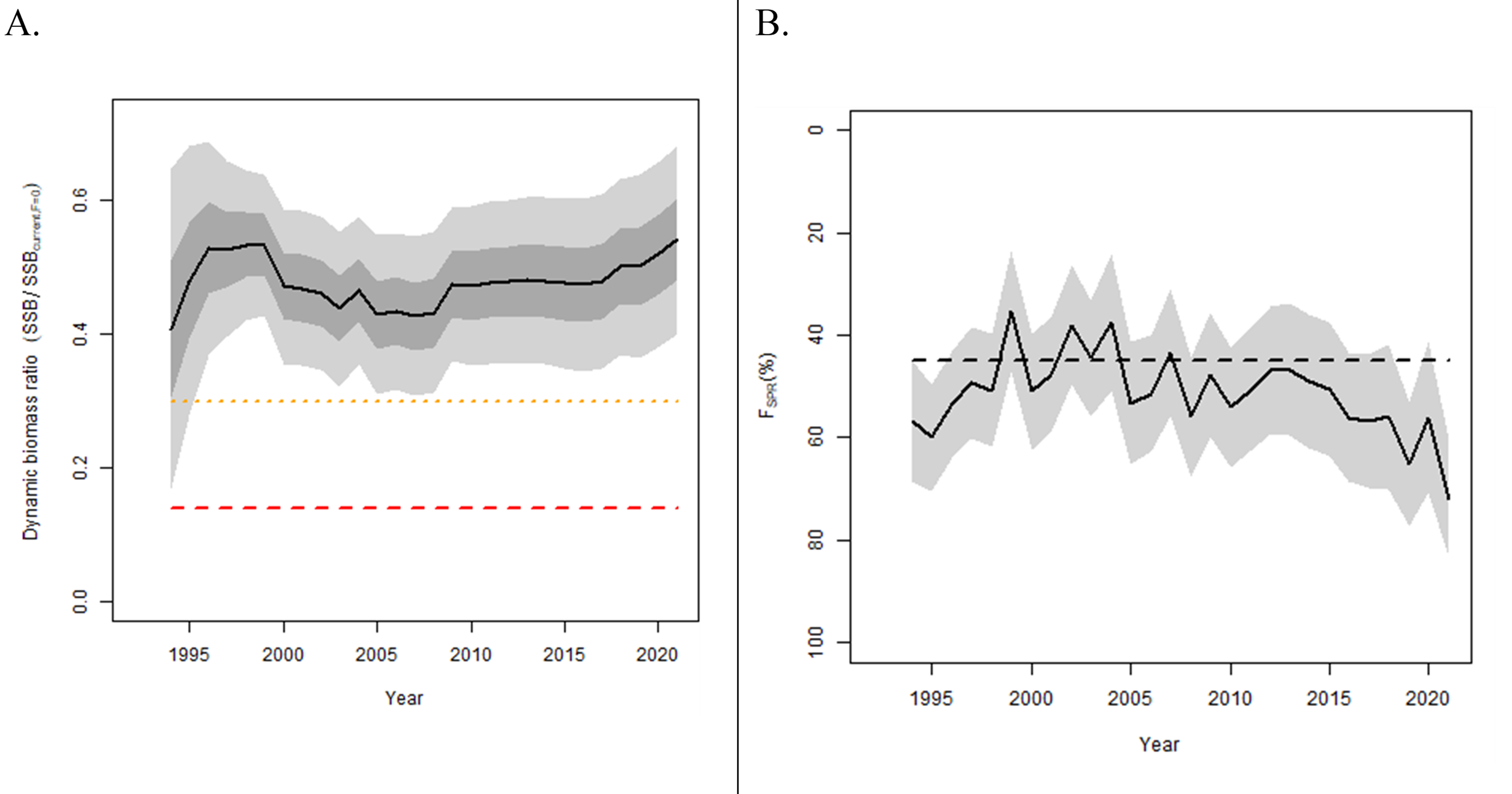Figure 6. (A) Estimated dynamic biomass ratio (SSB/SSBcurrent, F=0) of NPO ALB relative to biomass-based threshold (30%SSBcurrent, F=0) (orange dotted line) and limit (14%SSBcurrent, F=0) reference points (red dashed line) over the modeling period (1994 – 2021); and (B) estimated fishing intensity relative to the fishing intensity-based target reference point (F45%SPR) over the modeling period (1994 – 2021). Light and dark gray areas indicate 95% and 60% confidence intervals, respectively. The limit reference point is considered to be breached if the lower bound of the 60% confidence intervals overlaps the limit reference point.

Based on these findings, the following information on the status of the NPO ALB stock is provided by the ISC23 Plenary:

1. The stock is likely not overfished relative to the threshold (30%SSBcurrent, F=0) and limit (14%SSBcurrent, F=0) reference points adopted by the WCPFC and IATTC;
2. The stock is likely not experiencing overfishing relative to the adopted target reference point (F45%SPR); and
3. Current fishing intensity (F2018-2020) is lower than the average fishing intensity from the 2002-2004 period (the reference level for IATTC Resolution C-05-02 and WCPFC CMM-2019-03).

### Conservation Information

Two harvest scenarios were projected to evaluate impacts on achieving the management objectives for this stock, which are: 1) maintain SSB above the limit reference point, with a probability of at least 80% over the next 10 years; 2) maintain depletion of total biomass around the historical (2006-2015) average depletion over the next 10 years; and 3) maintain fishing intensity at or below the target reference point with a probability of at least 50% over the next 10 years (WCPFC HS 2022-01; IATTC Resolution C-22-04). As a larger cohort is estimated in the latest period of the assessment, all projections show a steep increase of SSB in the first year.

The constant fishing intensity scenario showed that at current fishing intensity (F2018-2020), female SSB is expected to increase to 90,098 t (95% CI: 23,218 – 156,978 t) by 2031. Over the next 10 years, there was: 1) a 97.7% probability of the female SSB remaining above the 14%SSBcurrent, F=0 LRP for all 10 years; 2) a 72.0% probability of the total biomass (age-1+) being above the average of 2006-2015 for any year; and 3) a 95.5% probability of the fishing intensity remaining at or below the F45%SPR TRP for any year (Figure 7).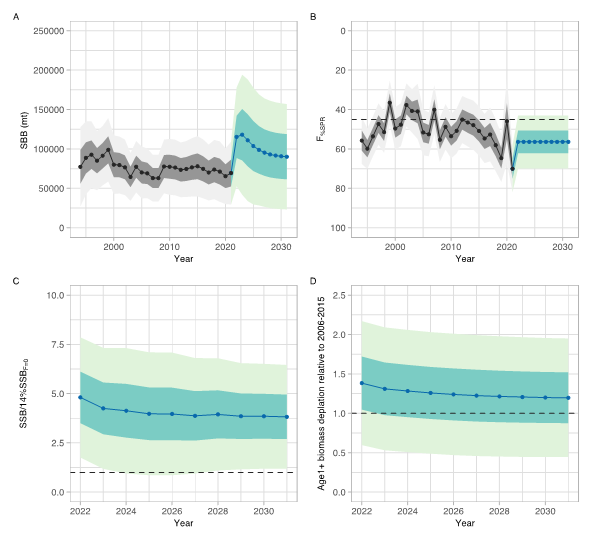Figure 7. Future projection results under a constant fishing intensity (F2018-2020) harvest scenario. Solid lines indicate mean values, uncertainty ranges indicate 60% and 95% confidence intervals, and the dashed line is the reference point, respectively. (A) Annual changes in spawning biomass; (B) Interannual changes in fishing mortality (F%SPR); (C) Projected ratios to the limit reference point thresholds; and (D) Projected ratios to management targets for the total biomass.

The randomly resampled fishing intensity scenario showed that at future fishing intensity similar to the 2005 – 2019 period, female SSB is expected to increase to 87,669 t (95% CI: 22,219 – 153,119 t) by 2031. Over the next 10 years, there was: 1) a 98.1 % probability of the female SSB remaining above the 14%SSBcurrent, F=0 LRP for all 10 years; 2) a 69.5 % probability of the total biomass (age-1+) being above the average of 2006 – 2015 for any year; and 3) a 79.6 % probability of the fishing intensity remaining at or below the F45%SPR TRP for any year (Figure 8).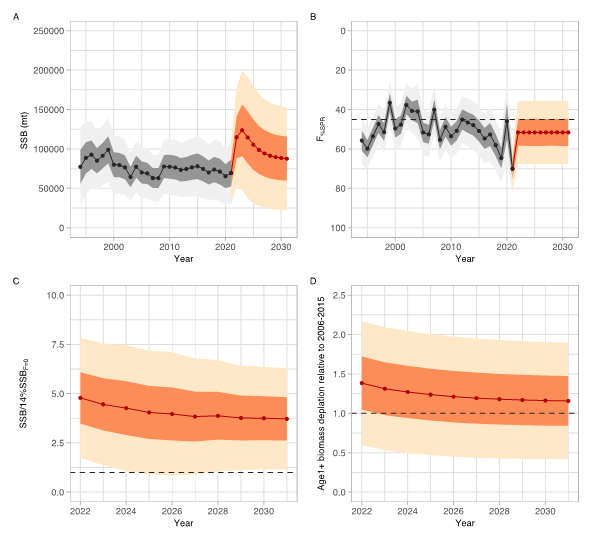Figure 8. Future projection results under a randomly sampled F (2005-2019) scenario. Solid lines indicate mean values, and uncertainty ranges indicate 60% and 95% confidence intervals, and the dashed line is the reference point, respectively. (A) Annual changes in spawning biomass; (B) Interannual changes in fishing mortality (F%SPR); (C) Projected ratios to the limit reference point thresholds; and (D) Projected ratios to management targets for the total biomass.

Based on these findings, the following conservation information is provided by the ISC23 Plenary for the NPO ALB stock:

1. If fishing intensity over the next ten years is maintained at the current fishing intensity (F2018-2020), then female SSB is expected to remain around 54%SSBcurrent,F=0 (90,098 t), with a 97.7% probability that female SSB will remain above the 14%SSBcurrent, F=0 LRP for all ten years and the management objectives of the IATTC and WCPFC will likely be met.
2. If fishing intensity over the next ten years is similar to the 2005 – 2019 period, then female SSB is expected to decrease to 52%SSBcurrent, F=0 (87,669 t), with a 98.1 % probability that female SSB will remain above the 14%SSBcurrent, F=0 LRP for all ten years and the management objectives of the IATTC and WCPFC will likely be met.